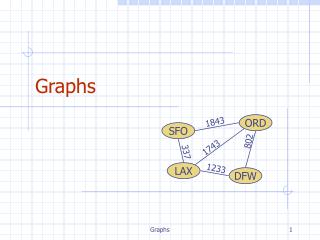Download PresentationGraphs

# Graphs - PowerPoint PPT PresentationDownload Presentation## Graphs

- - - - - - - - - - - - - - - - - - - - - - - - - - - E N D - - - - - - - - - - - - - - - - - - - - - - - - - - -
##### Presentation Transcript

1. Graphs 1843 ORD SFO 802 1743 337 1233 LAX DFW Graphs

2. Outline and Reading • Graphs (§6.1) • Definition • Applications • Terminology • Properties • ADT • Data structures for graphs (§6.2) • Edge list structure • Adjacency list structure • Adjacency matrix structure Graphs

3. A graph is a pair (V, E), where V is a set of nodes, called vertices E is a collection of pairs of vertices, called edges Vertices and edges are positions and store elements Example: A vertex represents an airport and stores the three-letter airport code An edge represents a flight route between two airports and stores the mileage of the route Graph 849 PVD 1843 ORD 142 SFO 802 LGA 1743 337 1387 HNL 2555 1099 1233 LAX 1120 DFW MIA Graphs

4. Edge Types • Directed edge • ordered pair of vertices (u,v) • first vertex u is the origin • second vertex v is the destination • e.g., a flight • Undirected edge • unordered pair of vertices (u,v) • e.g., a flight route • Directed graph • all the edges are directed • e.g., route network • Undirected graph • all the edges are undirected • e.g., flight network flight AA 1206 ORD PVD 849 miles ORD PVD Graphs

5. Applications • Electronic circuits • Printed circuit board • Integrated circuit • Transportation networks • Highway network • Flight network • Computer networks • Local area network • Internet • Web • Databases • Entity-relationship diagram Graphs

6. V a b h j U d X Z c e i W g f Y Terminology • End vertices (or endpoints) of an edge • U and V are the endpoints of a • Edges incident on a vertex • a, d, and b are incident on V • Adjacent vertices • U and V are adjacent • Degree of a vertex • X has degree 5 • Parallel edges • h and i are parallel edges • Self-loop • j is a self-loop Graphs

7. Terminology (cont.) • Path • sequence of alternating vertices and edges • begins with a vertex • ends with a vertex • each edge is preceded and followed by its endpoints • Simple path • path such that all its vertices and edges are distinct • Examples • P1=(V,b,X,h,Z) is a simple path • P2=(U,c,W,e,X,g,Y,f,W,d,V) is a path that is not simple V b a P1 d U X Z P2 h c e W g f Y Graphs

8. Terminology (cont.) • Cycle • circular sequence of alternating vertices and edges • each edge is preceded and followed by its endpoints • Simple cycle • cycle such that all its vertices and edges are distinct • Examples • C1=(V,b,X,g,Y,f,W,c,U,a,) is a simple cycle • C2=(U,c,W,e,X,g,Y,f,W,d,V,a,) is a cycle that is not simple V a b d U X Z C2 h e C1 c W g f Y Graphs

9. Notation n number of vertices m number of edges deg(v)degree of vertex v Property 1 Sv deg(v)= 2m Proof: each edge is counted twice Property 2 In an undirected graph with no self-loops and no multiple edges m  n (n -1)/2 Proof: each vertex has degree at most (n -1) What is the bound for a directed graph? Properties Example • n = 4 • m = 6 • deg(v)= 3 Graphs

10. Vertices and edges are positions store elements Accessor methods aVertex() incidentEdges(v) endVertices(e) isDirected(e) origin(e) destination(e) opposite(v, e) areAdjacent(v, w) Update methods insertVertex(o) insertEdge(v, w, o) insertDirectedEdge(v, w, o) removeVertex(v) removeEdge(e) Generic methods numVertices() numEdges() vertices() edges() Main Methods of the Graph ADT Graphs

11. Edge List Structure u • Vertex object • element • reference to position in vertex sequence • Edge object • element • origin vertex object • destination vertex object • reference to position in edge sequence • Vertex sequence • sequence of vertex objects • Edge sequence • sequence of edge objects a c b d v w z z w u v a b c d Graphs

12. Adjacency List Structure a v b • Edge list structure • Incidence sequence for each vertex • sequence of references to edge objects of incident edges • Augmented edge objects • references to associated positions in incidence sequences of end vertices u w w u v b a Graphs

13. Adjacency Matrix Structure a v b • Edge list structure • Augmented vertex objects • Integer key (index) associated with vertex • 2D-array adjacency array • Reference to edge object for adjacent vertices • Null for non nonadjacent vertices • The “old fashioned” version just has 0 for no edge and 1 for edge u w 2 w 0 u 1 v b a Graphs

14. Asymptotic Performance Graphs